Скачать презентацию Data Mining Concepts and Techniques Chapter 7

f350af2c73f229da1c3592987a13d957.ppt

• Количество слайдов: 36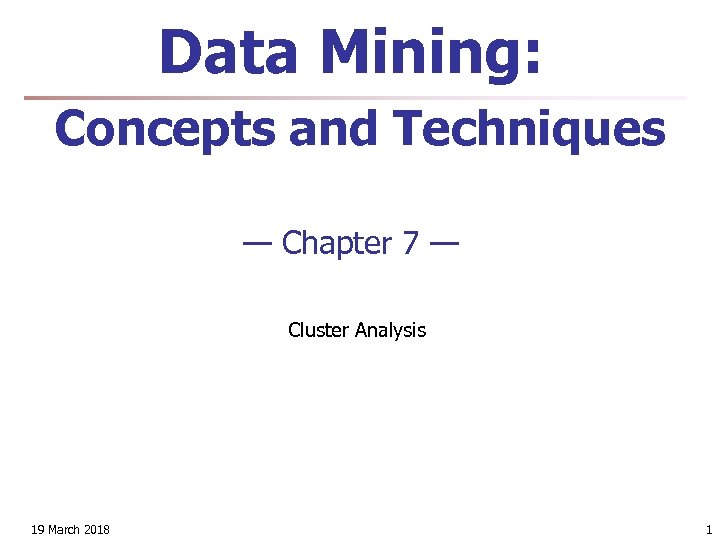Data Mining: Concepts and Techniques — Chapter 7 — Cluster Analysis 19 March 2018 1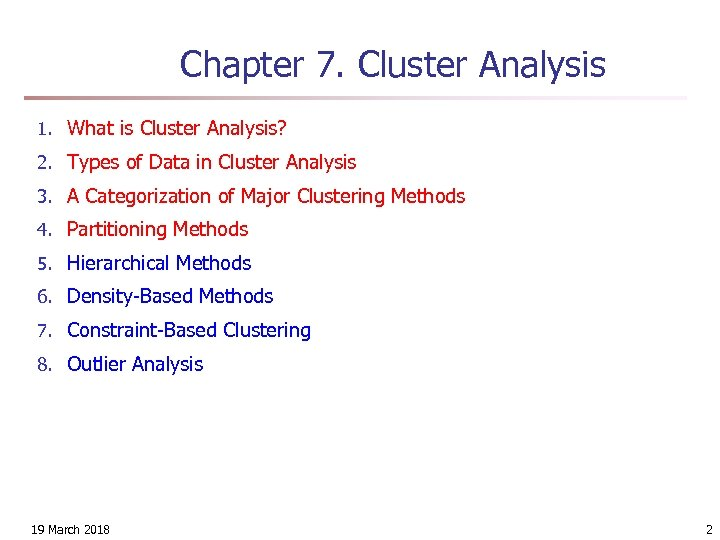Chapter 7. Cluster Analysis 1. What is Cluster Analysis? 2. Types of Data in Cluster Analysis 3. A Categorization of Major Clustering Methods 4. Partitioning Methods 5. Hierarchical Methods 6. Density-Based Methods 7. Constraint-Based Clustering 8. Outlier Analysis 19 March 2018 2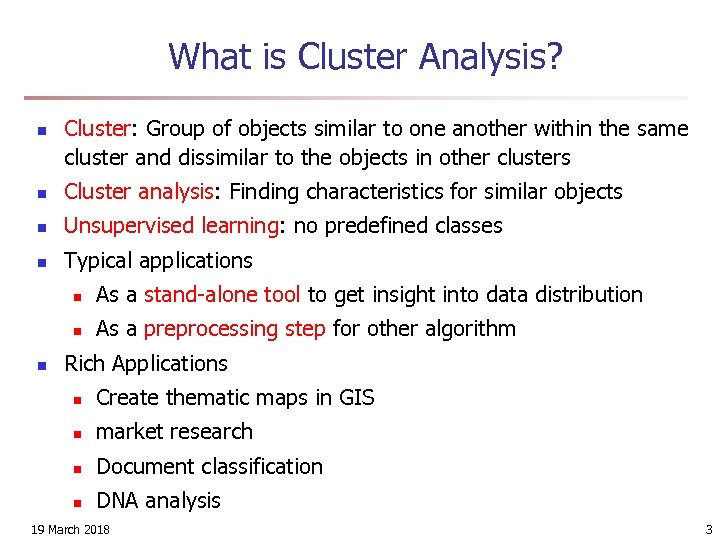What is Cluster Analysis? n Cluster: Group of objects similar to one another within the same cluster and dissimilar to the objects in other clusters n Cluster analysis: Finding characteristics for similar objects n Unsupervised learning: no predefined classes n Typical applications n n n As a stand-alone tool to get insight into data distribution As a preprocessing step for other algorithm Rich Applications n Create thematic maps in GIS n market research n Document classification n DNA analysis 19 March 2018 3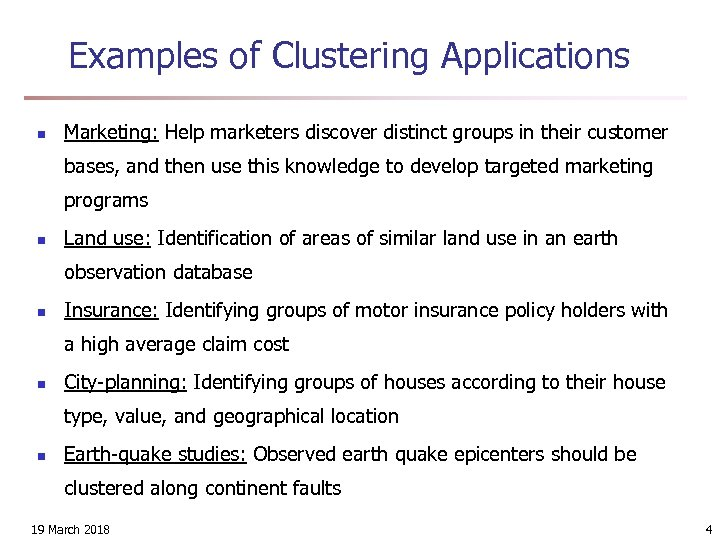Examples of Clustering Applications n Marketing: Help marketers discover distinct groups in their customer bases, and then use this knowledge to develop targeted marketing programs n Land use: Identification of areas of similar land use in an earth observation database n Insurance: Identifying groups of motor insurance policy holders with a high average claim cost n City-planning: Identifying groups of houses according to their house type, value, and geographical location n Earth-quake studies: Observed earth quake epicenters should be clustered along continent faults 19 March 2018 4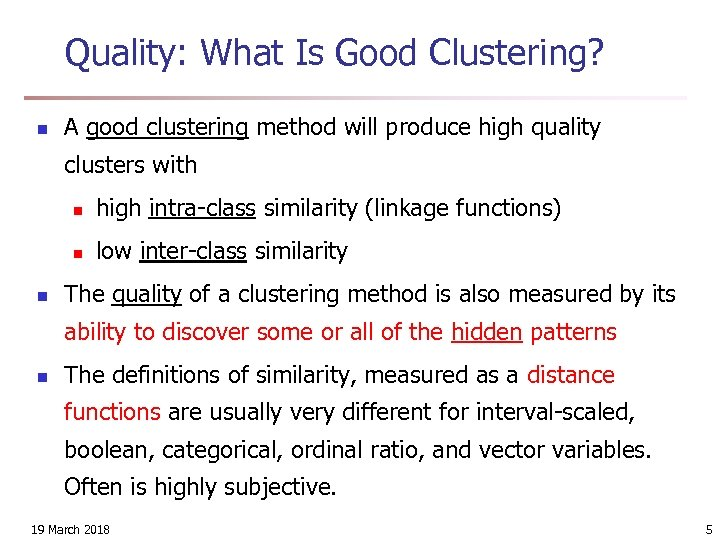Quality: What Is Good Clustering? n A good clustering method will produce high quality clusters with n n n high intra-class similarity (linkage functions) low inter-class similarity The quality of a clustering method is also measured by its ability to discover some or all of the hidden patterns n The definitions of similarity, measured as a distance functions are usually very different for interval-scaled, boolean, categorical, ordinal ratio, and vector variables. Often is highly subjective. 19 March 2018 5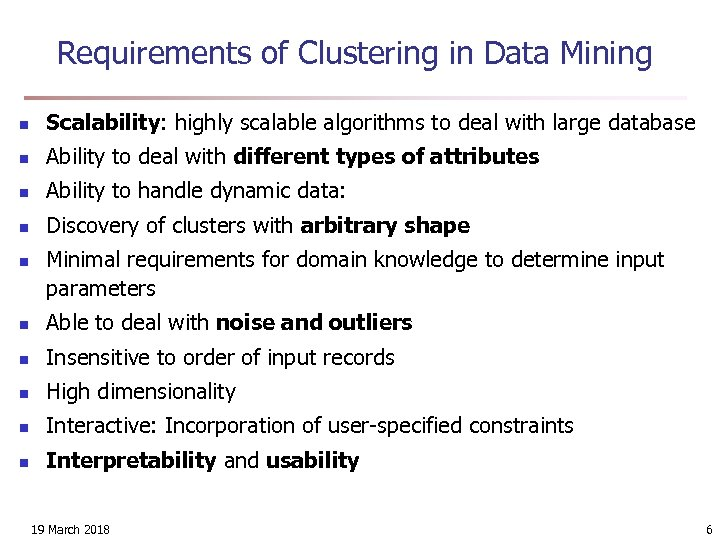Requirements of Clustering in Data Mining n Scalability: highly scalable algorithms to deal with large database n Ability to deal with different types of attributes n Ability to handle dynamic data: n Discovery of clusters with arbitrary shape n Minimal requirements for domain knowledge to determine input parameters n Able to deal with noise and outliers n Insensitive to order of input records n High dimensionality n Interactive: Incorporation of user-specified constraints n Interpretability and usability 19 March 2018 6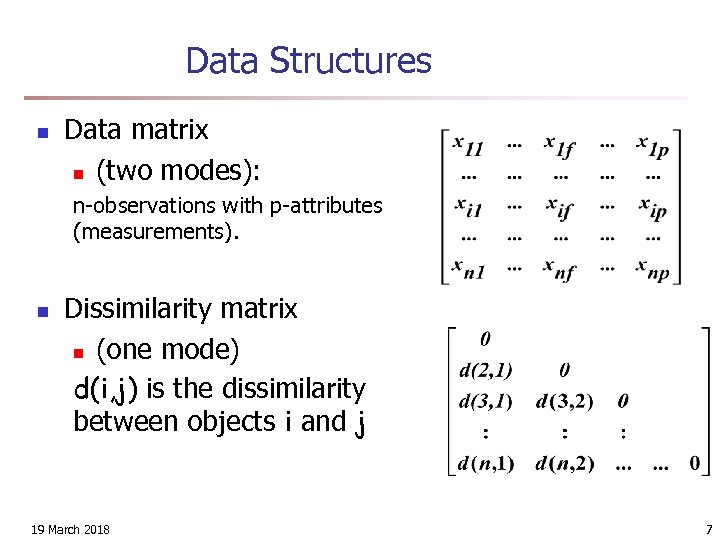Data Structures n Data matrix n (two modes): n-observations with p-attributes (measurements). n Dissimilarity matrix n (one mode) d(i, j) is the dissimilarity between objects i and j 19 March 2018 7Type of data in clustering analysis n Interval-scaled variables ( continuous measures) n Binary variables n Nominal, ordinal, and ratio variables n Variables of mixed types 19 March 2018 8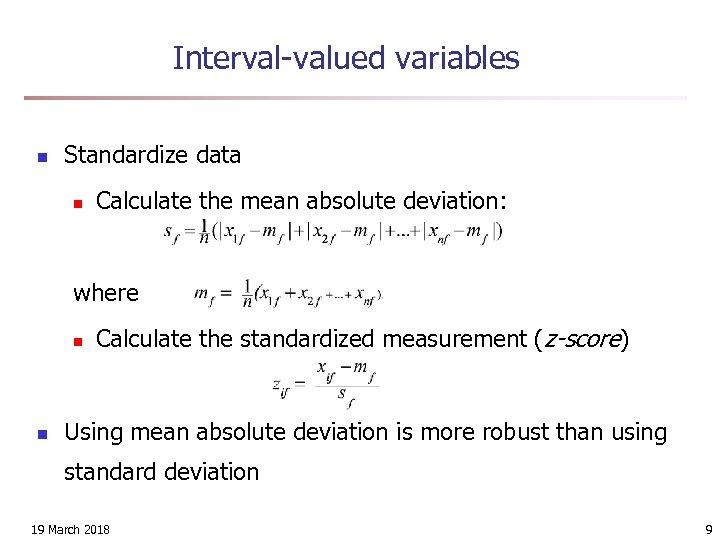Interval-valued variables n Standardize data n Calculate the mean absolute deviation: where n n Calculate the standardized measurement (z-score) Using mean absolute deviation is more robust than using standard deviation 19 March 2018 9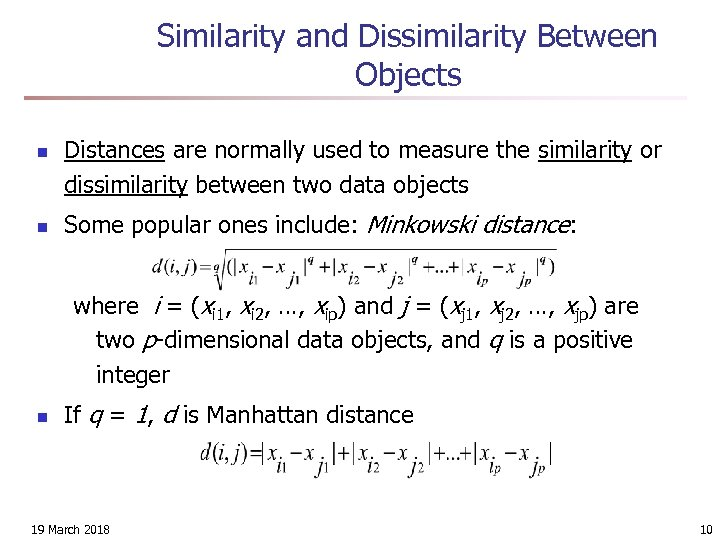Similarity and Dissimilarity Between Objects n n Distances are normally used to measure the similarity or dissimilarity between two data objects Some popular ones include: Minkowski distance: where i = (xi 1, xi 2, …, xip) and j = (xj 1, xj 2, …, xjp) are two p-dimensional data objects, and q is a positive integer n If q = 1, d is Manhattan distance 19 March 2018 10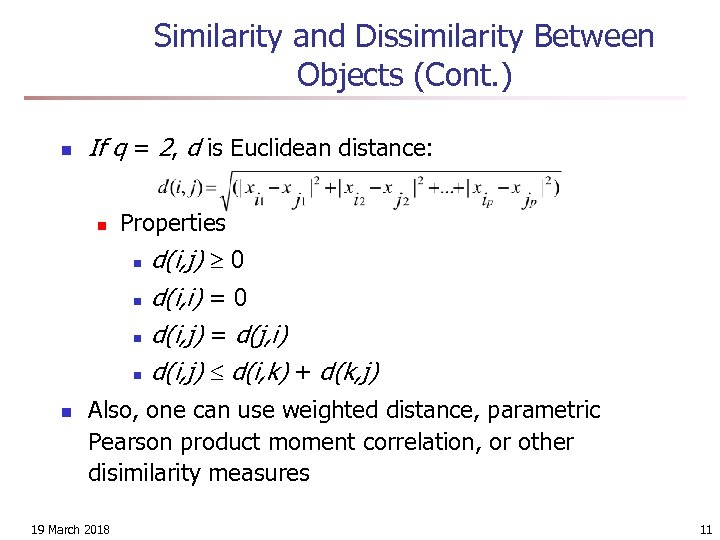Similarity and Dissimilarity Between Objects (Cont. ) n If q = 2, d is Euclidean distance: n Properties n n n d(i, j) 0 d(i, i) = 0 d(i, j) = d(j, i) d(i, j) d(i, k) + d(k, j) Also, one can use weighted distance, parametric Pearson product moment correlation, or other disimilarity measures 19 March 2018 11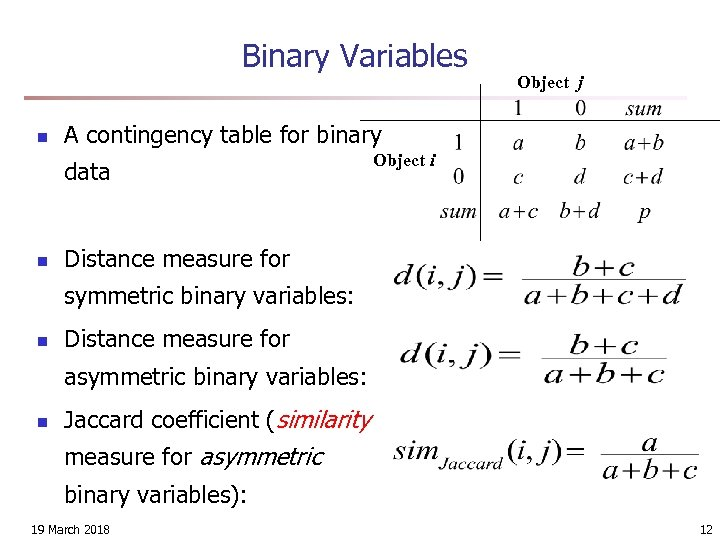Binary Variables n A contingency table for binary data n Object j Object i Distance measure for symmetric binary variables: n Distance measure for asymmetric binary variables: n Jaccard coefficient (similarity measure for asymmetric binary variables): 19 March 2018 12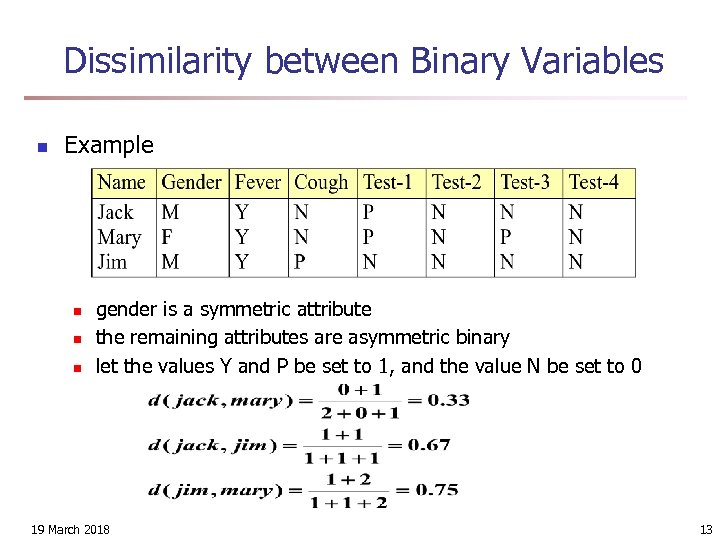Dissimilarity between Binary Variables n Example n n n gender is a symmetric attribute the remaining attributes are asymmetric binary let the values Y and P be set to 1, and the value N be set to 0 19 March 2018 13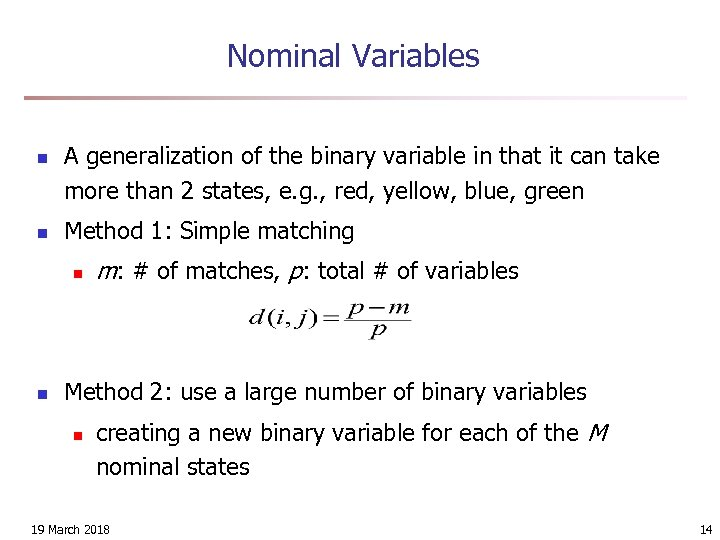Nominal Variables n n A generalization of the binary variable in that it can take more than 2 states, e. g. , red, yellow, blue, green Method 1: Simple matching n n m: # of matches, p: total # of variables Method 2: use a large number of binary variables n creating a new binary variable for each of the M nominal states 19 March 2018 14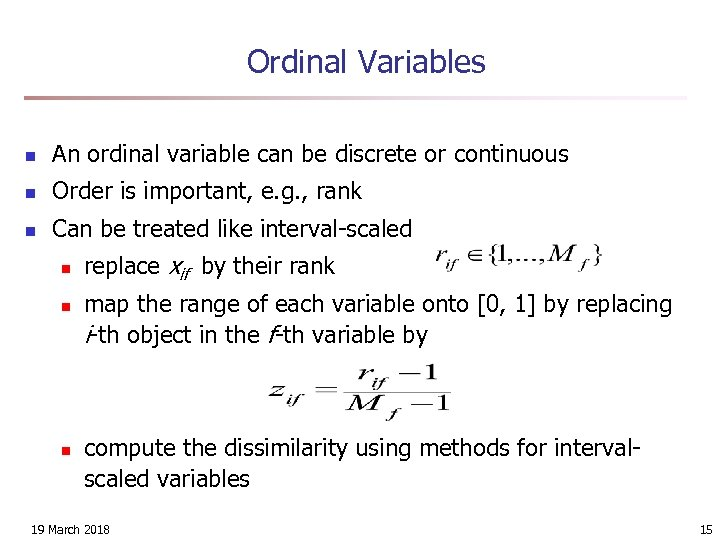Ordinal Variables n An ordinal variable can be discrete or continuous n Order is important, e. g. , rank n Can be treated like interval-scaled n n n replace xif by their rank map the range of each variable onto [0, 1] by replacing i-th object in the f-th variable by compute the dissimilarity using methods for intervalscaled variables 19 March 2018 15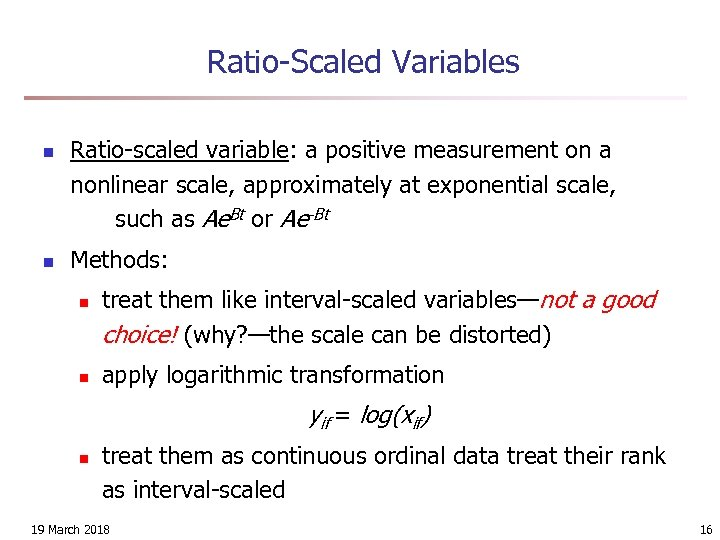Ratio-Scaled Variables n n Ratio-scaled variable: a positive measurement on a nonlinear scale, approximately at exponential scale, such as Ae. Bt or Ae-Bt Methods: n n treat them like interval-scaled variables—not a good choice! (why? —the scale can be distorted) apply logarithmic transformation yif = log(xif) n treat them as continuous ordinal data treat their rank as interval-scaled 19 March 2018 16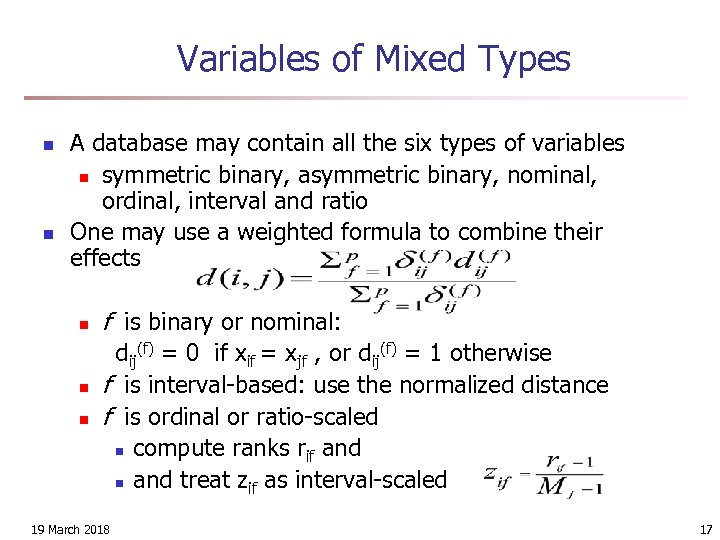Variables of Mixed Types n n A database may contain all the six types of variables n symmetric binary, asymmetric binary, nominal, ordinal, interval and ratio One may use a weighted formula to combine their effects n n n f is binary or nominal: dij(f) = 0 if xif = xjf , or dij(f) = 1 otherwise f is interval-based: use the normalized distance f is ordinal or ratio-scaled n compute ranks rif and n and treat zif as interval-scaled 19 March 2018 17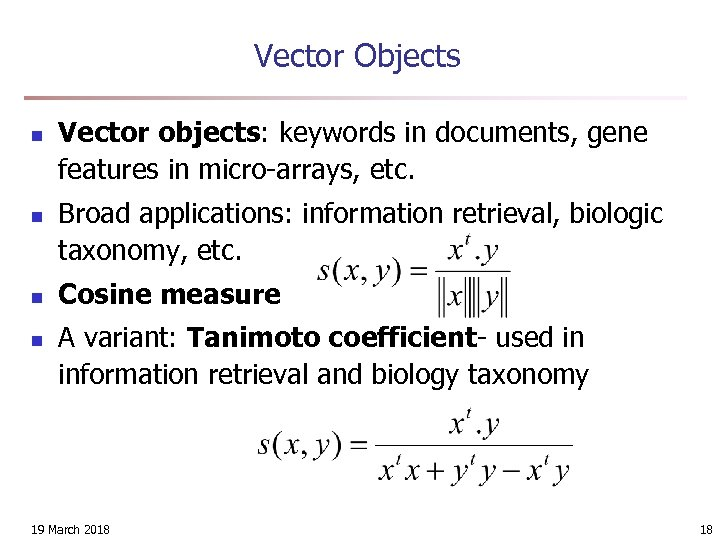Vector Objects n n Vector objects: keywords in documents, gene features in micro-arrays, etc. Broad applications: information retrieval, biologic taxonomy, etc. Cosine measure A variant: Tanimoto coefficient- used in information retrieval and biology taxonomy 19 March 2018 18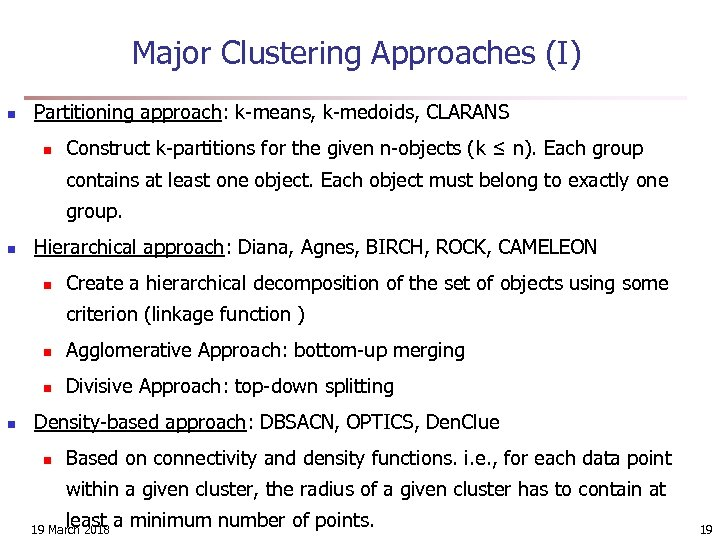Major Clustering Approaches (I) n Partitioning approach: k-means, k-medoids, CLARANS n Construct k-partitions for the given n-objects (k ≤ n). Each group contains at least one object. Each object must belong to exactly one group. n Hierarchical approach: Diana, Agnes, BIRCH, ROCK, CAMELEON n Create a hierarchical decomposition of the set of objects using some criterion (linkage function ) n n n Agglomerative Approach: bottom-up merging Divisive Approach: top-down splitting Density-based approach: DBSACN, OPTICS, Den. Clue n Based on connectivity and density functions. i. e. , for each data point within a given cluster, the radius of a given cluster has to contain at least a minimum number of points. 19 March 2018 19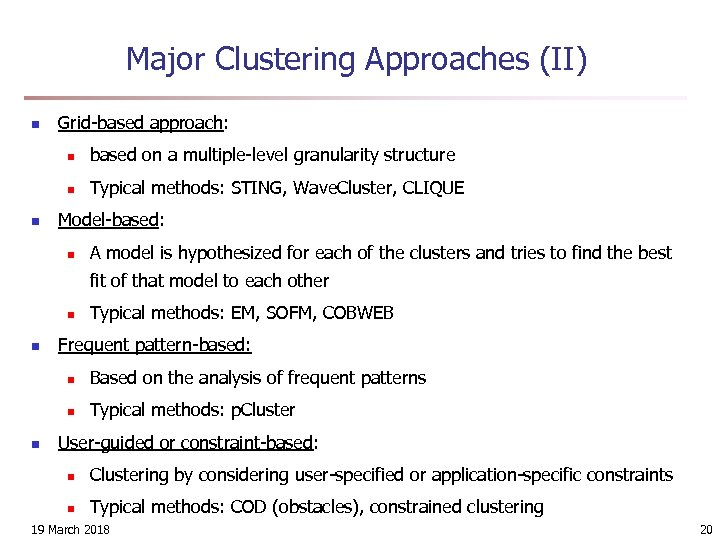Major Clustering Approaches (II) n Grid-based approach: n n n based on a multiple-level granularity structure Typical methods: STING, Wave. Cluster, CLIQUE Model-based: n A model is hypothesized for each of the clusters and tries to find the best fit of that model to each other n n Typical methods: EM, SOFM, COBWEB Frequent pattern-based: n n n Based on the analysis of frequent patterns Typical methods: p. Cluster User-guided or constraint-based: n Clustering by considering user-specified or application-specific constraints n Typical methods: COD (obstacles), constrained clustering 19 March 2018 20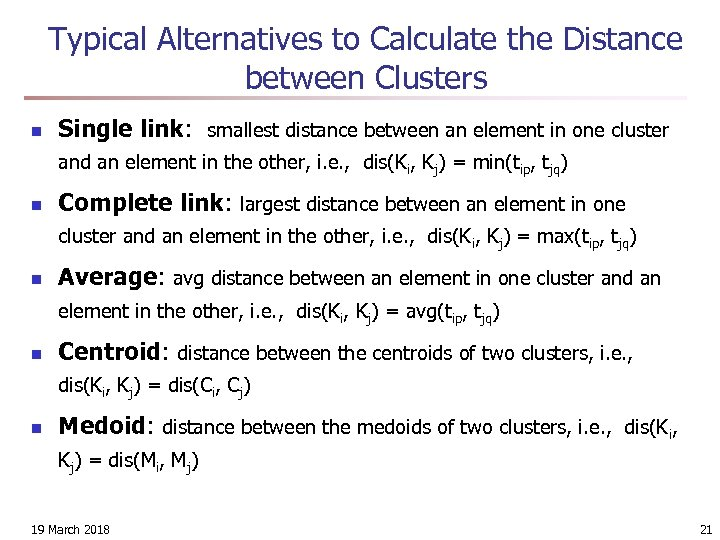Typical Alternatives to Calculate the Distance between Clusters n Single link: smallest distance between an element in one cluster and an element in the other, i. e. , dis(Ki, Kj) = min(tip, tjq) n Complete link: largest distance between an element in one cluster and an element in the other, i. e. , dis(Ki, Kj) = max(tip, tjq) n Average: avg distance between an element in one cluster and an element in the other, i. e. , dis(Ki, Kj) = avg(tip, tjq) n Centroid: distance between the centroids of two clusters, i. e. , dis(Ki, Kj) = dis(Ci, Cj) n Medoid: distance between the medoids of two clusters, i. e. , dis(Ki, Kj) = dis(Mi, Mj) 19 March 2018 21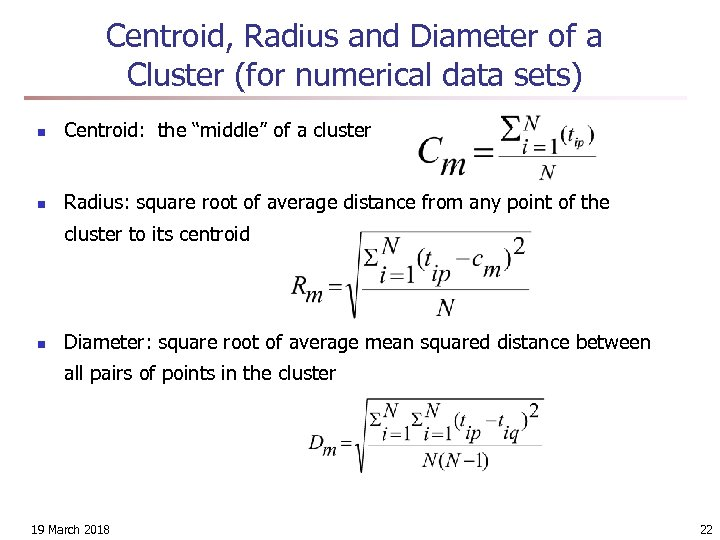Centroid, Radius and Diameter of a Cluster (for numerical data sets) n Centroid: the “middle” of a cluster n Radius: square root of average distance from any point of the cluster to its centroid n Diameter: square root of average mean squared distance between all pairs of points in the cluster 19 March 2018 22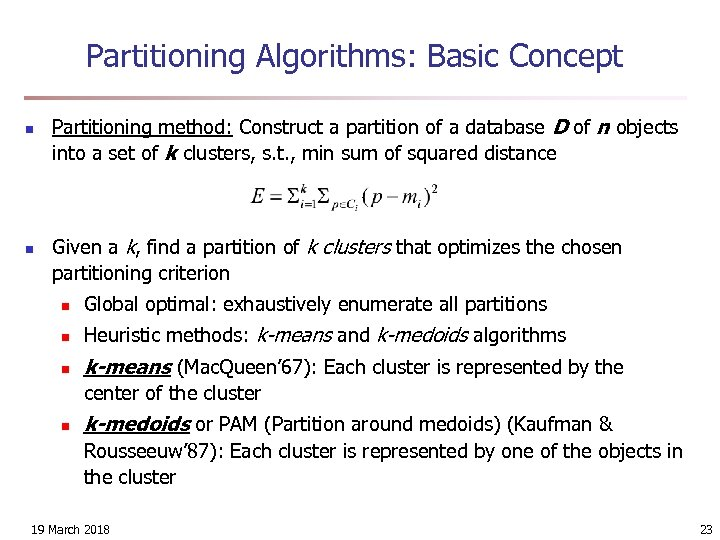Partitioning Algorithms: Basic Concept n n Partitioning method: Construct a partition of a database D of n objects into a set of k clusters, s. t. , min sum of squared distance Given a k, find a partition of k clusters that optimizes the chosen partitioning criterion n Global optimal: exhaustively enumerate all partitions n Heuristic methods: k-means and k-medoids algorithms n k-means (Mac. Queen’ 67): Each cluster is represented by the center of the cluster n k-medoids or PAM (Partition around medoids) (Kaufman & Rousseeuw’ 87): Each cluster is represented by one of the objects in the cluster 19 March 2018 23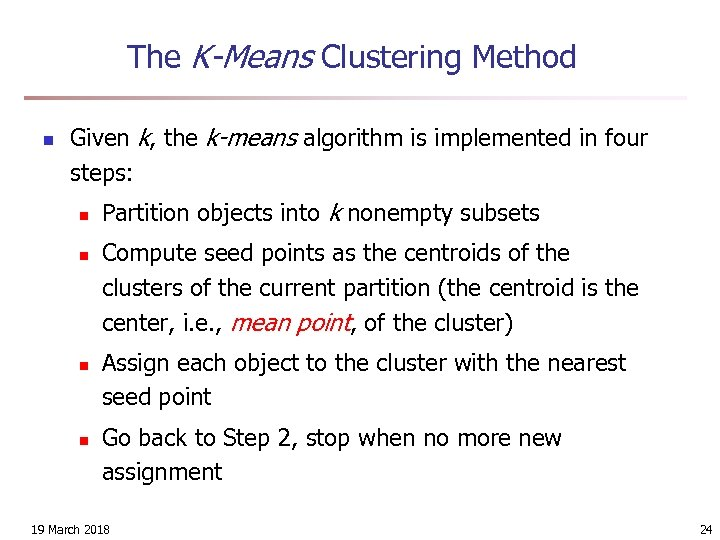The K-Means Clustering Method n Given k, the k-means algorithm is implemented in four steps: n n Partition objects into k nonempty subsets Compute seed points as the centroids of the clusters of the current partition (the centroid is the center, i. e. , mean point, of the cluster) Assign each object to the cluster with the nearest seed point Go back to Step 2, stop when no more new assignment 19 March 2018 24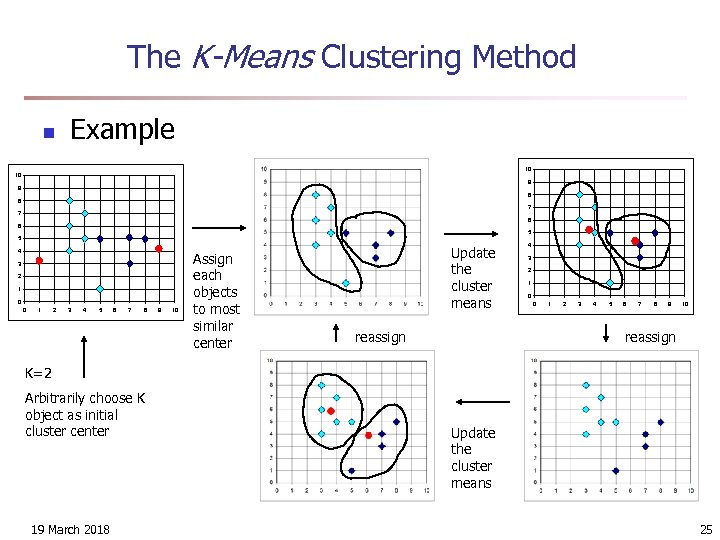The K-Means Clustering Method n Example 10 10 9 9 8 8 7 7 6 6 5 5 4 3 2 1 0 0 1 2 3 4 5 6 7 8 9 10 Assign each objects to most similar center Update the cluster means reassign 4 3 2 1 0 0 1 2 3 4 5 6 7 8 9 10 reassign K=2 Arbitrarily choose K object as initial cluster center 19 March 2018 Update the cluster means 25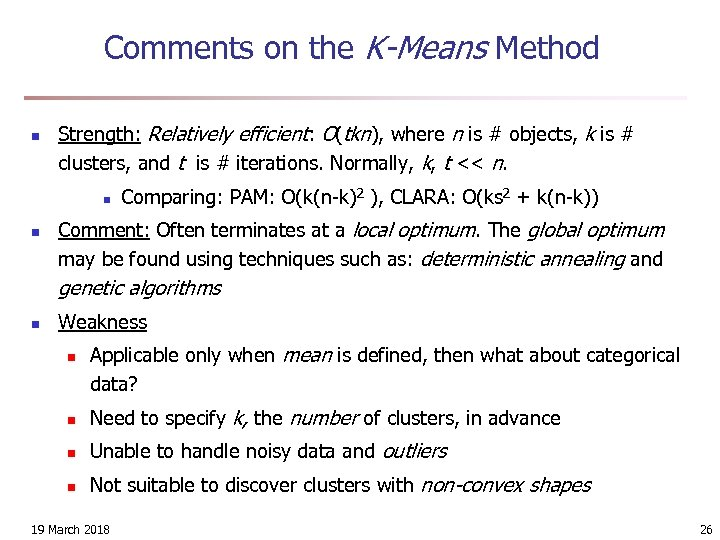Comments on the K-Means Method n Strength: Relatively efficient: O(tkn), where n is # objects, k is # clusters, and t is # iterations. Normally, k, t << n. n n Comparing: PAM: O(k(n-k)2 ), CLARA: O(ks 2 + k(n-k)) Comment: Often terminates at a local optimum. The global optimum may be found using techniques such as: deterministic annealing and genetic algorithms n Weakness n Applicable only when mean is defined, then what about categorical data? n Need to specify k, the number of clusters, in advance n Unable to handle noisy data and outliers n Not suitable to discover clusters with non-convex shapes 19 March 2018 26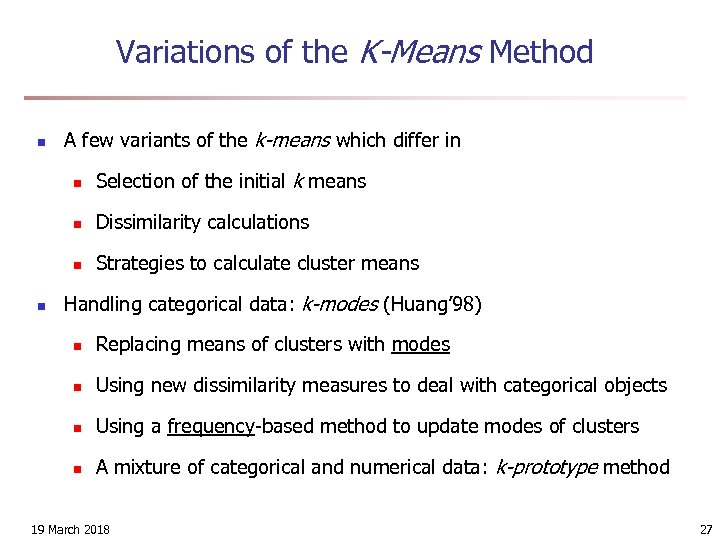Variations of the K-Means Method n A few variants of the k-means which differ in n n Dissimilarity calculations n n Selection of the initial k means Strategies to calculate cluster means Handling categorical data: k-modes (Huang’ 98) n Replacing means of clusters with modes n Using new dissimilarity measures to deal with categorical objects n Using a frequency-based method to update modes of clusters n A mixture of categorical and numerical data: k-prototype method 19 March 2018 27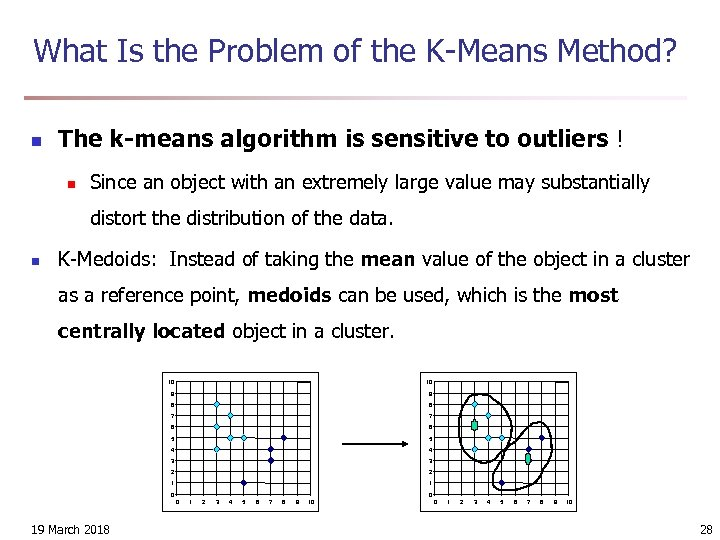What Is the Problem of the K-Means Method? n The k-means algorithm is sensitive to outliers ! n Since an object with an extremely large value may substantially distort the distribution of the data. n K-Medoids: Instead of taking the mean value of the object in a cluster as a reference point, medoids can be used, which is the most centrally located object in a cluster. 10 10 9 9 8 8 7 7 6 6 5 5 4 4 3 3 2 2 1 1 0 0 0 19 March 2018 1 2 3 4 5 6 7 8 9 10 0 1 2 3 4 5 6 7 8 9 10 28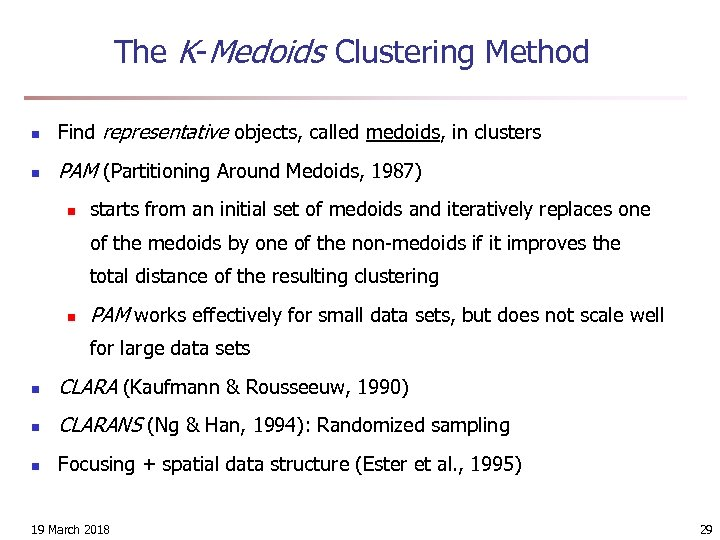The K-Medoids Clustering Method n Find representative objects, called medoids, in clusters n PAM (Partitioning Around Medoids, 1987) n starts from an initial set of medoids and iteratively replaces one of the medoids by one of the non-medoids if it improves the total distance of the resulting clustering n PAM works effectively for small data sets, but does not scale well for large data sets n CLARA (Kaufmann & Rousseeuw, 1990) n CLARANS (Ng & Han, 1994): Randomized sampling n Focusing + spatial data structure (Ester et al. , 1995) 19 March 2018 29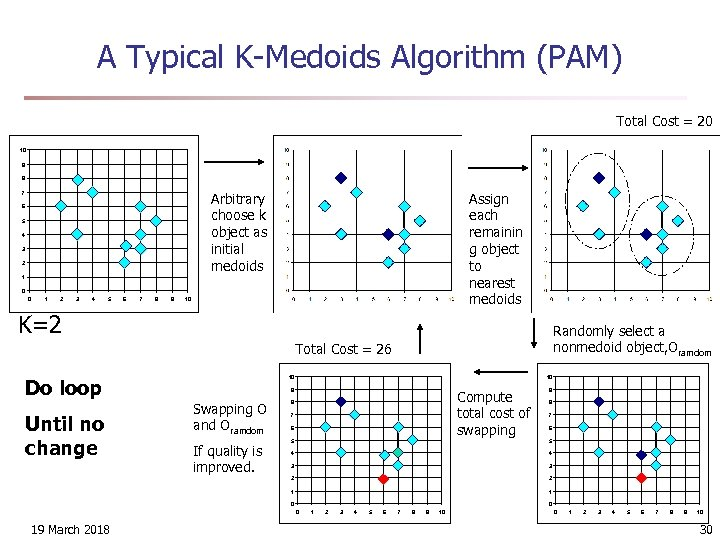A Typical K-Medoids Algorithm (PAM) Total Cost = 20 10 9 8 Arbitrary choose k object as initial medoids 7 6 5 4 3 2 Assign each remainin g object to nearest medoids 1 0 0 1 2 3 4 5 6 7 8 9 10 K=2 Randomly select a nonmedoid object, Oramdom Total Cost = 26 Do loop Until no change 10 10 9 Swapping O and Oramdom Compute total cost of swapping 8 7 6 9 8 7 6 5 4 4 3 3 2 2 1 If quality is improved. 5 1 0 0 0 19 March 2018 1 2 3 4 5 6 7 8 9 10 0 1 2 3 4 5 6 7 8 9 10 30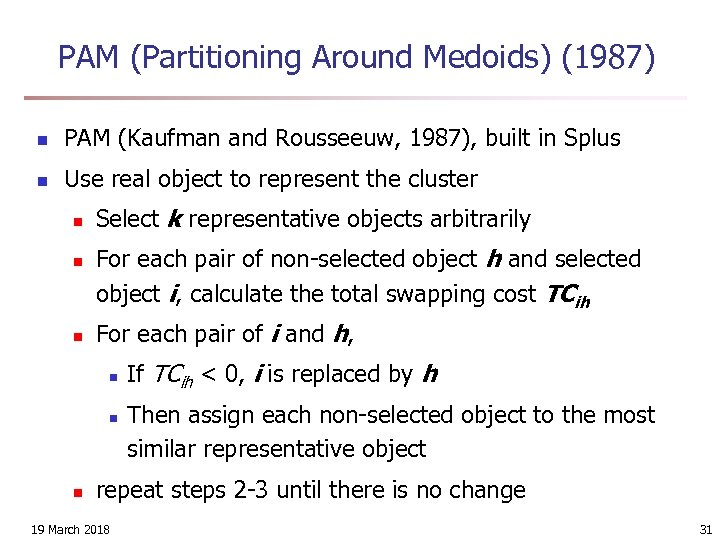PAM (Partitioning Around Medoids) (1987) n PAM (Kaufman and Rousseeuw, 1987), built in Splus n Use real object to represent the cluster n n n Select k representative objects arbitrarily For each pair of non-selected object h and selected object i, calculate the total swapping cost TCih For each pair of i and h, n n n If TCih < 0, i is replaced by h Then assign each non-selected object to the most similar representative object repeat steps 2 -3 until there is no change 19 March 2018 31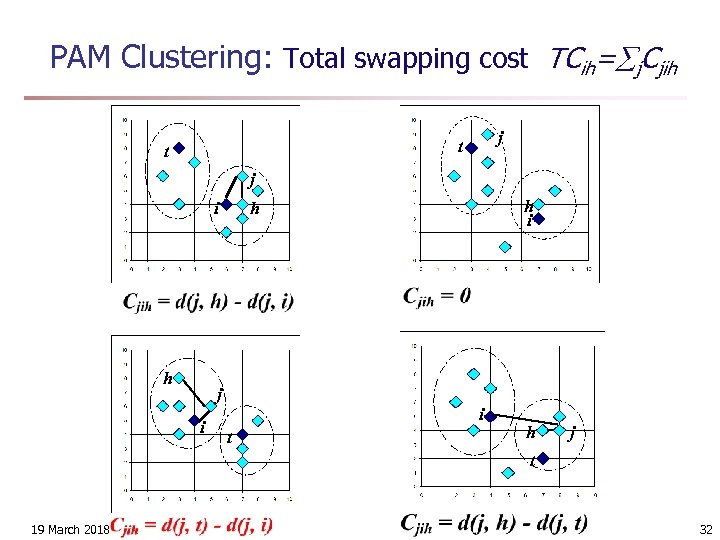PAM Clustering: Total swapping cost TCih= j. Cjih j t t j i h i h i t h j t 19 March 2018 32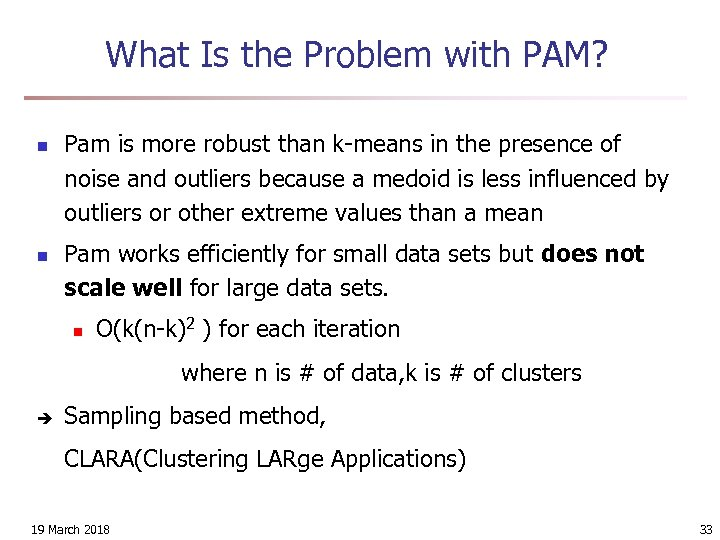What Is the Problem with PAM? n n Pam is more robust than k-means in the presence of noise and outliers because a medoid is less influenced by outliers or other extreme values than a mean Pam works efficiently for small data sets but does not scale well for large data sets. n O(k(n-k)2 ) for each iteration where n is # of data, k is # of clusters è Sampling based method, CLARA(Clustering LARge Applications) 19 March 2018 33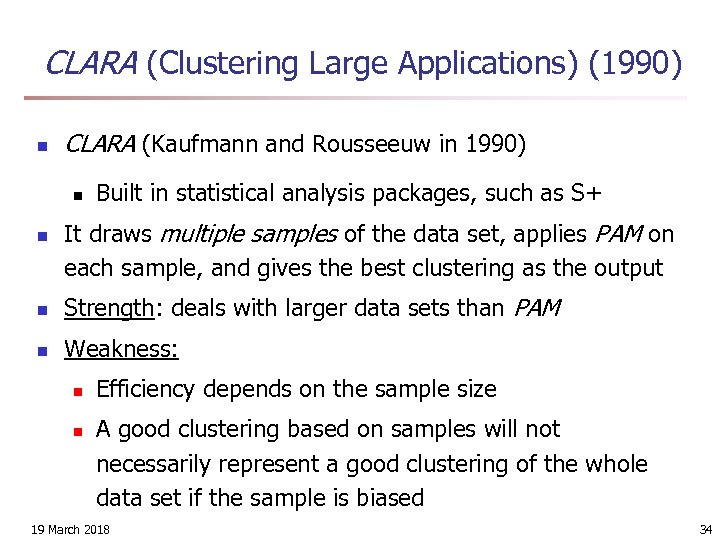CLARA (Clustering Large Applications) (1990) n CLARA (Kaufmann and Rousseeuw in 1990) n n Built in statistical analysis packages, such as S+ It draws multiple samples of the data set, applies PAM on each sample, and gives the best clustering as the output n Strength: deals with larger data sets than PAM n Weakness: n n Efficiency depends on the sample size A good clustering based on samples will not necessarily represent a good clustering of the whole data set if the sample is biased 19 March 2018 34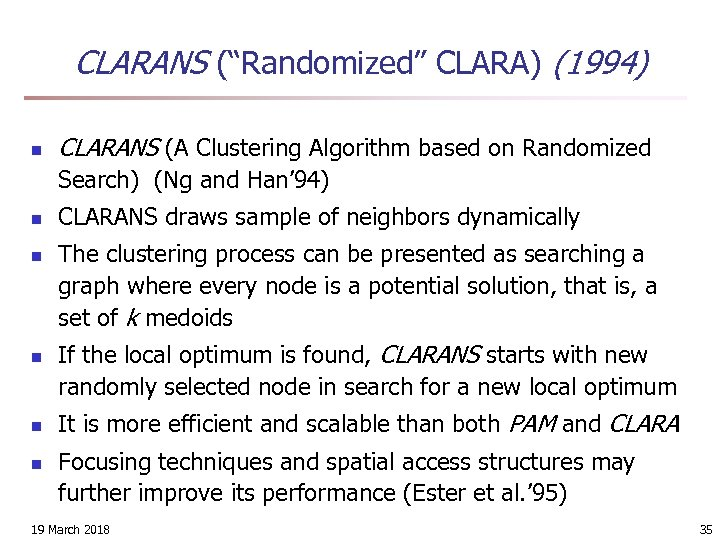CLARANS (“Randomized” CLARA) (1994) n CLARANS (A Clustering Algorithm based on Randomized Search) (Ng and Han’ 94) n n n CLARANS draws sample of neighbors dynamically The clustering process can be presented as searching a graph where every node is a potential solution, that is, a set of k medoids If the local optimum is found, CLARANS starts with new randomly selected node in search for a new local optimum It is more efficient and scalable than both PAM and CLARA Focusing techniques and spatial access structures may further improve its performance (Ester et al. ’ 95) 19 March 2018 35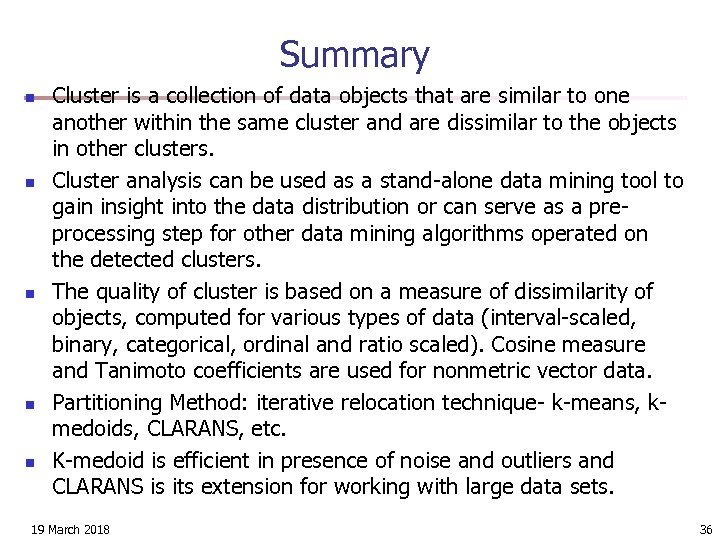Summary n n n Cluster is a collection of data objects that are similar to one another within the same cluster and are dissimilar to the objects in other clusters. Cluster analysis can be used as a stand-alone data mining tool to gain insight into the data distribution or can serve as a preprocessing step for other data mining algorithms operated on the detected clusters. The quality of cluster is based on a measure of dissimilarity of objects, computed for various types of data (interval-scaled, binary, categorical, ordinal and ratio scaled). Cosine measure and Tanimoto coefficients are used for nonmetric vector data. Partitioning Method: iterative relocation technique- k-means, kmedoids, CLARANS, etc. K-medoid is efficient in presence of noise and outliers and CLARANS is its extension for working with large data sets. 19 March 2018 36